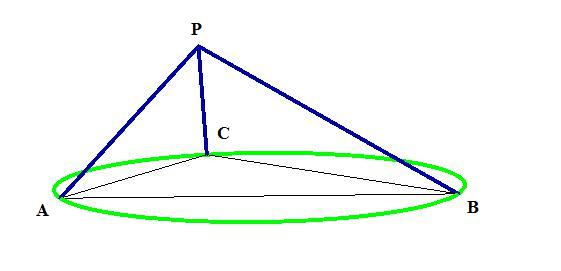# Trirectangular Corner Locus

Given a circle of radius $1$, the locus of all $P$ such that there are points $A, B, C$ on the circle such that$\angle APB=\angle BPC=\angle CPA=90°$

is a surface of a solid that has a volume $V$. Find $\left\lfloor 100V \right\rfloor$

This is a 3D generalization of the fact that the locus of all $P$ on a circle makes a right triangle with the diameter.

×